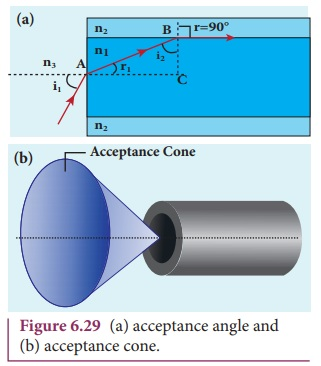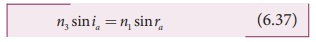Home | | Physics 12th Std | Effects due to total internal reflection

# Effects due to total internal reflection

1. Glittering of diamond 2. Mirage and looming 3. Prisms making using of total internal reflection 4. Radius of illumination (Snell’s window) 5. Optical fiber 6. Acceptance angle in optical fibre

Effects due to total internal reflection

### 1. Glittering of diamond

Diamond appears dazzling because the total internal reflection of light happens inside the diamond. The refractive index of only diamond is about 2.417. It is much larger than that for ordinary glass which is about only 1.5. The critical angle of diamond is about 24.4°. It is much less than that of glass. A skilled diamond cutter makes use of this larger range of angle of incidence (24.4° to 90° inside the diamond), to ensure that light entering the diamond is total internally reflected from the many cut faces before getting out as shown in Figure 6.22. This gives a sparkling effect for diamond.### 2. Mirage and looming

The refractive index of air increases with its density. In hot places, air near the ground is hotter than air at a height. Hot air is less dense. Hence, in still air the refractive index of air increases with height. Because of this, light from tall objects like a tree, passes through a medium whose refractive index decreases towards the ground. Hence, a ray of light successively deviates away from the normal at different layers of air and undergoes total internal reflection when the angle of incidence near the ground exceeds the critical angle. This gives an illusion as if the light comes from somewhere below the ground. For of the shaky nature of the layers of air, the observer feels as if the object is getting reflected by a pool of water or wet surface beneath the object as shown in Figure 6.23(a). This phenomenon is called mirage.

In the cold places the refractive index increases towards the ground because the temperature of air close to the ground is lesser than the temperature above the surface of earth. Thus, the density and refractive index of air near the ground is greater than at a height. In the cold regions like glaciers and frozen lakes and seas, the reverse effect of mirage will happen. Hence, an inverted image is formed little above the surface as shown in Figure 6.23(b). This phenomenon is called looming.### 3. Prisms making using of total internal reflection

Prisms can be designed to reflect light by 90º or by 180º by making use of total internal reflection as shown in Figure 6.24(a) and (b). In the first two cases, the critical angle ic for the material of the prism must be less than 45º. We see from Table 6.3 that this is true for both crown glass and flint glass. Prisms are also used to invert images without changing their size as shown in Figure. 6.24(c).### 4. Radius of illumination (Snell’s window)When a light source like electric bulb is kept inside a water tank, the light from the source travels in all direction inside the water. The light that is incident on the water surface at an angle less than the critical angle will undergo refraction and emerge out from the water. The light incident at an angle greater than critical angle will undergo total internal reflection.The light falling particularly at critical angle graces the surface.Thus, the entire surface of water appears illuminated when seen from outside as shown in Figure 6.25.

On the other hand, when light entering the water from outside is seen from inside the water, the view is restricted to a particular angle equal to the critical angle ic. The restricted illuminated circular area is called Snell’s window as shown in Figure 6.26(a).The Figure 6.26(b) shows the angle of view for water animals.The angle of view for water animals is restricted to twice the critical angle 2ic. The critical angle for water is 48.6º. Thus the angle of view is 97.2 º. The radius R of the circular area depends on the depth d from which it is seen and also the refractive indices of the  media. The radius of Snell’s window can be deduced with the illustration as shown in Figure 6.27.Light is seen from a point A at a depth d. The Snell’s law in product form, equation (6.19) for the refraction happening at the point B on the boundary between the two media is,Equating the above two equation 6.34 and equation 6.35,If the rarer medium outside is air, then, n2 = 1, and we can take n1=n### EXAMPLE 6.9

What is the radius of the illumination when seen above from inside a swimming pool from a depth of 10 m on a sunny day? What is the total angle of view? [Given, refractive index of water is 4/3]

Solution

Given, n = 4/3, d = 10 m.The total angle of view is,

2ic = 2 × 48.6º =  97.2º

### 5. Optical fiberTransmitting signals through optical fibres is possible due to the phenomenon of total internal reflection. Optical fibres consists of inner part called core and outer part called cladding (or) sleeving. The refractive index of the material of the core must be higher than that of the cladding for total internal reflection to happen. Signal in the form of light is made to incident inside the core-cladding boundary at an angle greater than the critical angle. Hence, it undergoes repeated total internal reflections along the length of the fibre without undergoing any refraction. The light travels inside the core with no appreciable loss in the intensity of the light as shown in Figure 6.28(a). Even while bending the optic fiber, it is done in such a way that the condition for total internal reflection is ensured at every reflection as shown in Figure 6.28(b).

### 6. Acceptance angle in optical fibre

To ensure the critical angle incidence in the core-cladding boundary inside the optical fibre, the light should be incident at a certain angle at the end of the optical fiber while entering in to it. This angle is called acceptance angle. It depends on the refractive indices of the core n1, cladding n2 and the outer medium n3. Assume the light is incident at an angle called acceptance angle ia at the outer medium and core boundary at A.The Snell’s law in the product form, equation (6.19) for this refraction at the point A is as shown in the Figure 6.29(a),To have the total internal reflection inside optical fibre, the angle of incidence at the core-cladding interface at B should be atleast critical angle ic. Snell’s law in the product form, equation (6.19) for the refraction at point B is,If outer medium is air, then n3 = 1. The acceptance angle ia becomes,Light can have any angle of incidence from 0 to ia with the normal at the end of the optical fibre forming a conical shape called acceptance cone as shown in Figure 6.29(b). In the equation (6.42), the term (n3 sin ia) is called numerical aperture NA of the optical fibre.If outer medium is air, then n3= 1. The numerical aperture NA becomes,### EXAMPLE 6.10

A optical fibre is made up of a core material with refractive index 1.68 and a cladding material of refractive index 1.44. What is the acceptance angle of the fibre kept in air medium? What is the answer if there is no cladding?

### Solution

Given, n1 = 1.68, n2 = 1.44, n1 = 1sin−1 (more than 1) is not possible. But, this includes the range 0º to 90º. Hence, all the rays entering the core from flat surface will undergo total internal reflection.

Note: If there is no cladding then there is a condition on the refractive index (n1) of the core.

ia = sin−1(√[n12 −1])

Here, as per mathematical rule, (n2 −1) ≤ 1 or (n12 )≤ 2

or n1 ≤ √2

Hence, in air (no cladding) the refractive index n1 of the core should be, n1 ≤ 1.414

An endoscope is an instrument used by doctors which has a bundle of optical fibres that are used to see inside a patient’s body. Endoscopes work on the phenomenon of total internal reflection. The optical fibres are inserted in to the body through mouth, nose or a special hole made in the body. Even operations could be carried out with the endoscope cable which has the necessary instruments attached at their ends.Tags : Optics | Physics , 12th Physics : UNIT 6 : Ray Optics
Study Material, Lecturing Notes, Assignment, Reference, Wiki description explanation, brief detail
12th Physics : UNIT 6 : Ray Optics : Effects due to total internal reflection | Optics | Physics